January 21, 2018

## What are species distribution models (SDMs)?

"Species distribution models (SDMs) are numerical tools that combine observations of species occurrence or abundance with environmental estimates. They are used to gain ecological and evolutionary insights and to predict distributions across landscapes, sometimes requiring extrapolation in space and time." – Elith & Leathwick 2009

Elith, J. and Leathwick, J.R., 2009. Species distribution models: ecological explanation and prediction across space and time. Annual review of ecology, evolution, and systematics, 40, pp.677-697.

## Types of SDMs

• Type of statistical models
• Frequentist
• Bayesian
• Machine learning

## Types of SDMs, cont'd

• Type of response variables
• Presence/absence (occurrence)
• Count (abundance)
• Number of response variables
• One
• single species
• Uni-directional interspecific interactions (B -> A)
• Two or more: multiple species
• Interspecific similarity
• Undirectional interspecific interactions (A - B)
• Bi-directional interspecific interactions (A -> B & B -> A)

## Types of SDMs, cont'd

• Temporal processes represented
• No: static
• Yes: dynamic
• Spatial processes represented
• No
• Implicitly (spatial autocorrelation)
• Explicitly (dynamic)

## Basic frequetist SDMs (that no one uses)

• Occurrence: logistic regression $y \sim Bernoulli(\pi)$ $logit(\pi) = X\beta$

• Abundance: Poisson regression $N \sim Poisson(\lambda)$ $log(\lambda)=X\beta$

## Two directions of extension

Machine learning (non-parametric) Bayesian (parametric)
Non-linear relationship Easy to detect Hard to detect
Observation error Hard to account Easy to account

## Today's focus

• Occurrence
• Single species
• Static, non-spatial

## Two issues to consider

• Non-linear relationships
• Observation error

## Two approaches for one goal

• Multivariate adaptive regression spline (MARS)
• Detect non-linear relationship
• Occupancy model
• Account for detection error

## What is MARS

• Developed by Friedman (Friedman, J.H., 1991. Multivariate adaptive regression splines. The annals of statistics, pp.1-67.)
• Connect a bunch of straight segment to represent non-linear relationships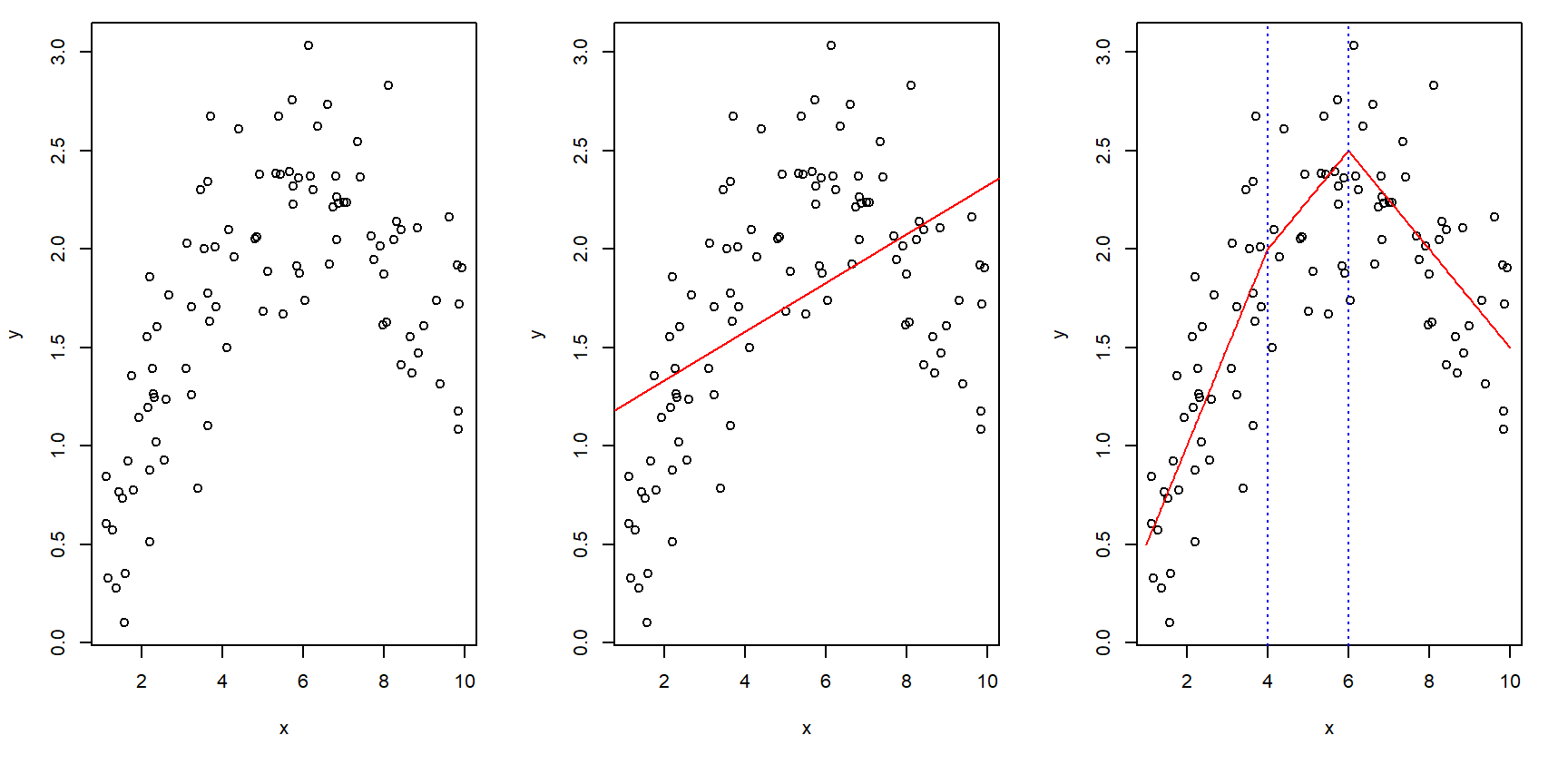## What are occupancy models

• Developed by MacKenzie and colleagues (MacKenzie, D.I., Nichols, J.D., Lachman, G.B., Droege, S., Andrew Royle, J. and Langtimm, C.A., 2002. Estimating site occupancy rates when detection probabilities are less than one. Ecology, 83(8), pp.2248-2255.)
• It is a hierarchical Bayesian model that includes an observation sub-model and a process sub-model

Observation:
$y_{i,j} \sim Binomial(z_{i} \times p, J_{i})$ Process: $z_{i} \sim Bernoulli(\pi_{i})$ $logit(\pi_{i}) = x^T \beta$

## Case study

• Lion beetle in Alberta, Canada
• Relate lion beetle occurrence with temperature and forest cover
• Predict lion beetle distribution

## Study area and survey sites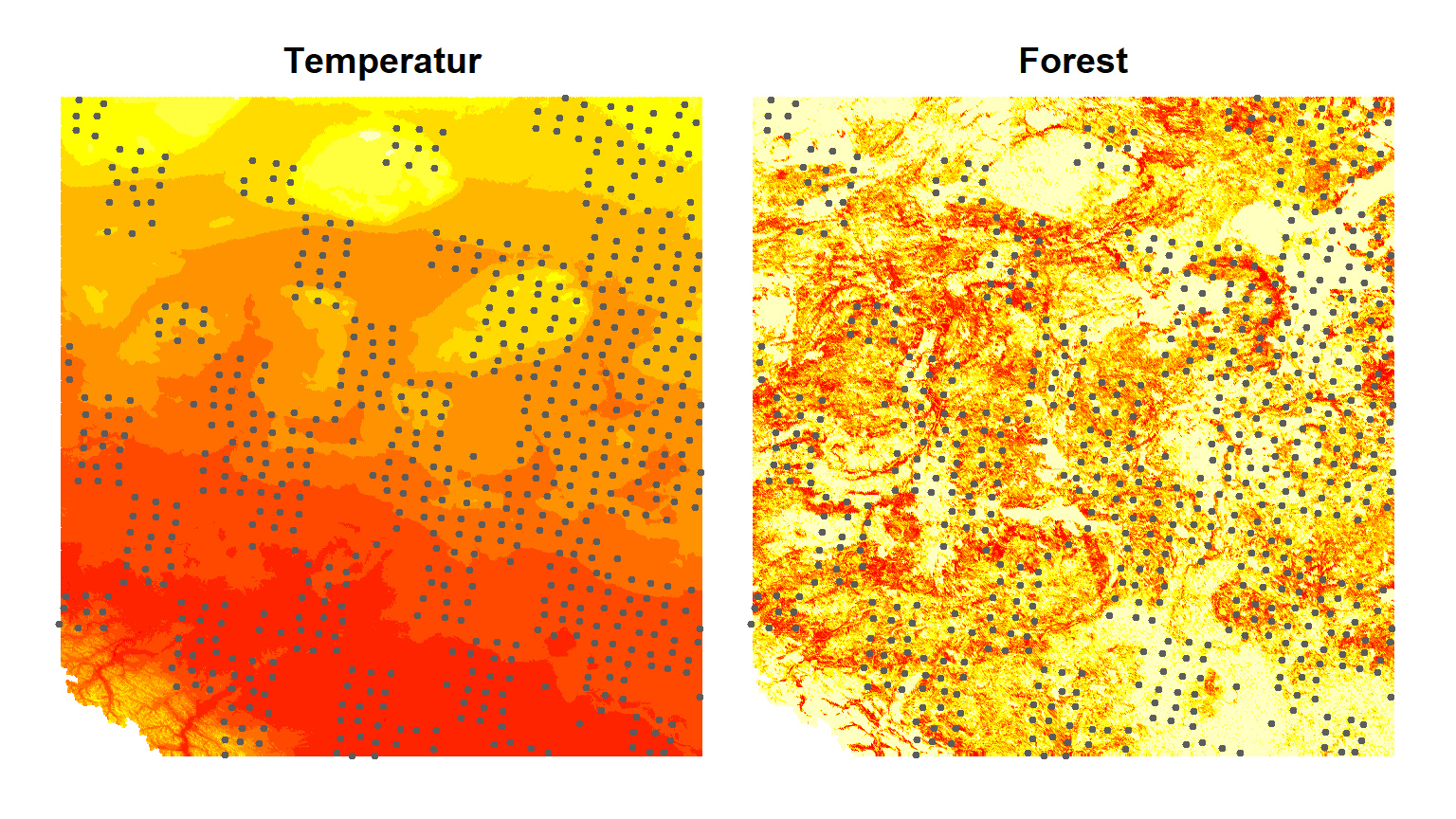## Look at the data

load('c:/A. UFL/rmeetup/rmeetup data.RData')
head(data, n=3)
##   y1 y2 y3 y4 temperature    forest
## 1  0  0  0  0   -2.432446 1.0000000
## 2  0  1  0  0    1.127675 0.0000000
## 3  1  1  1  1    1.127675 0.7039379

## Things can get complicated quickly

Distribution-environment relationship Model
Forest
Temperature + Forest
Forest ^ 2
Temperature ^ 2 + Forest
Temperature + Forest ^ 2
Temperature ^ 2 + Forest ^ 2

## And more

Distribution-environment relationship Model
Interaction Temperature * Forest
(Temperature ^ 2) * Forest
Temperature * (Forest ^ 2)
(Temperature ^ 2) * (Forest ^ 2)

## Use MARS to make things easier

library(earth) # This is the library for MARS
# Create "occupancy" data for MARS
data$y01 <- ifelse(rowSums(data[,1:4])==0, 0, 1) head(data, n=3) ## y1 y2 y3 y4 temperature forest y01 ## 1 0 0 0 0 -2.432446 1.0000000 0 ## 2 0 1 0 0 1.127675 0.0000000 1 ## 3 1 1 1 1 1.127675 0.7039379 1 ## Use MARS to analyze the data # Model one, only additive relationships are considered fit1 <- earth(y01 ~ temperature + forest, data=data, glm=list(family='binomial'), # for occurrence data degree=1, # for additive model pmethod='backward', nfold=20, keepxy=TRUE) # for k-fold cross validation # Model two, consider interactions between covariates fit2 <- earth(y01 ~ temperature + forest, data=data, glm=list(family='binomial'), degree=2, # include level-one interactions pmethod='backward', nfold=20, keepxy=TRUE) ## Model comparison Look at the AUC values of cross-validation auc <- cbind(fit1$cv.auc.tab[1:nfold,1], fit2$cv.auc.tab[1:nfold,1], boxplot(auc, axes=F, ylim=c(0,1), xlab='Model', ylab='AUC') axis(side=1, at=1:2, labels=c('Temp + Forest','Temp x Forest')) axis(side=2, at=seq(0,1,.5)) box()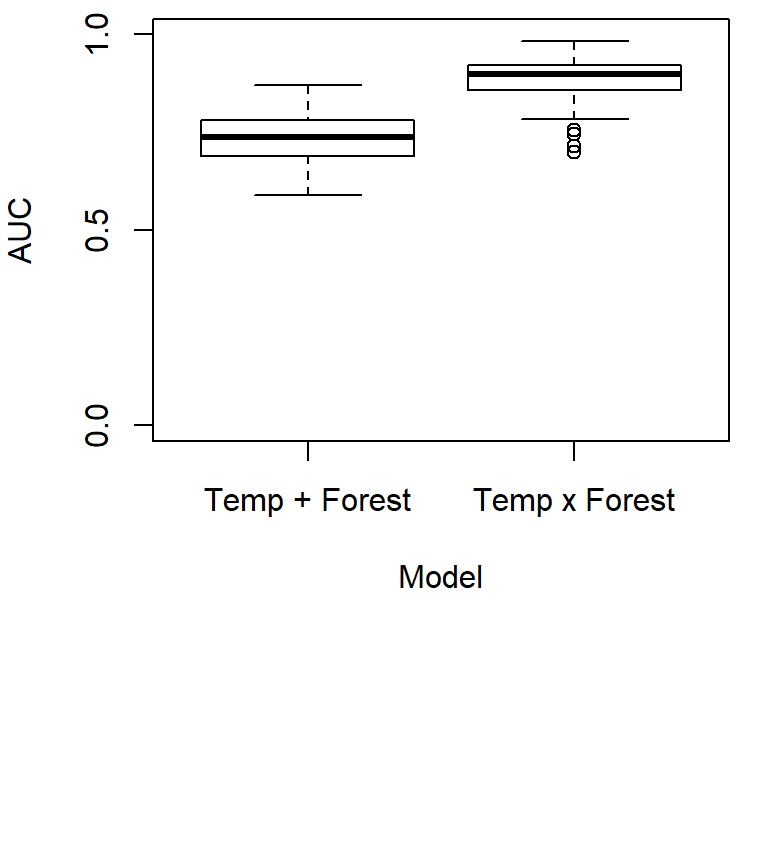## The importance of covariates evimp(fit2) ## variable nsubsets gcv rss ## 1 temperature 13 100.0 100.0 ## 2 forest 11 47.8 51.7 ## Look at the response curves npred <- 100 forest.highlow <- c(0, 1) ypred <- matrix(0, npred, 2) for (i in 1:2) { xpred <- cbind(seq(-3, 3, length.out=npred), rep(forest.highlow[i], npred)) ypred[,i] <- inv.logit(predict(object=fit2, newdata=xpred)) } xseq <- seq(-3, 3, length.out=npred) par(mfrow=c(1,1)) par(mar=c(5,5,1,1)) plot(ypred[,1] ~ xseq, type='n', ylim=c(0,1), xlab='Temperature', ylab='Prob. of Occu.') for (i in 1:2) {lines(ypred[,i] ~ xseq, col=2^i)} legend('topright', bty='n', lty=1, col=2^c(1:2), legend=c('low','high'), title='Forest')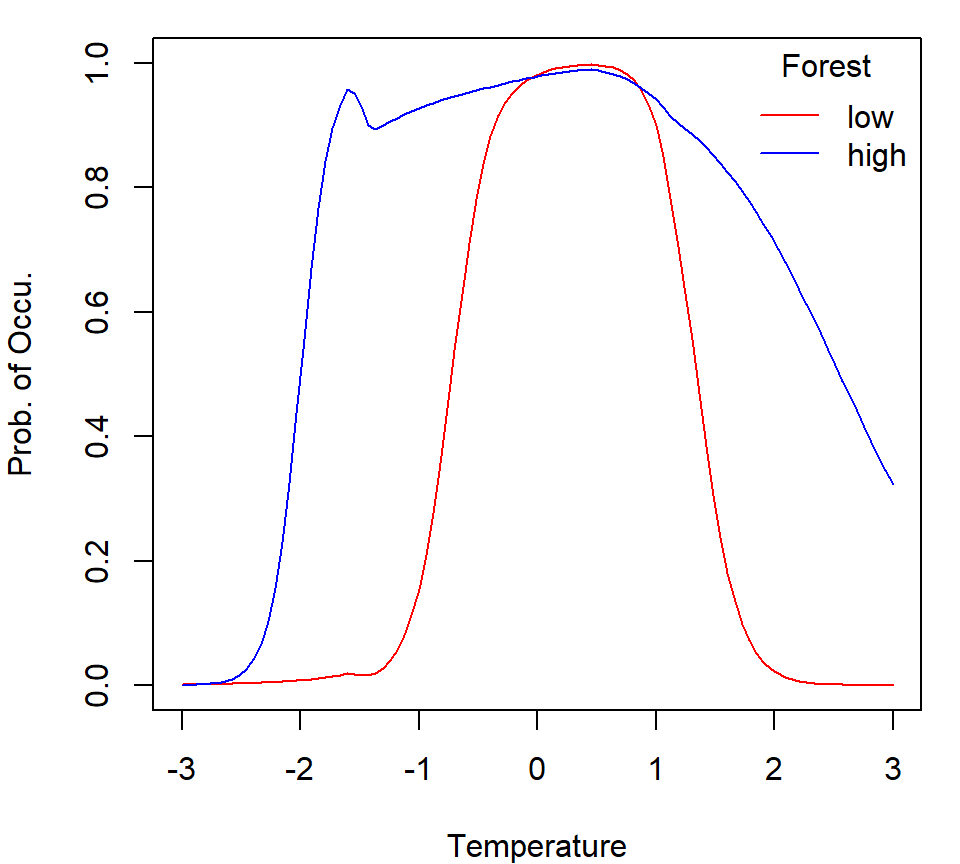## What we learned from MARS • An interaction between temperature and forest is needed • A quandratic form of temperature is needed ## Issues yet to solve • Observation error ## Conduct occupancy modeling library(unmarked) # This is the library for occupancy models # Prepare data for "unmarked" unmarked.data <- unmarkedFrameOccu(y=data[,1:4], siteCovs=data[,c('temperature','forest')], obsCovs=NULL) ## Run "unmarked" to fit the MacKenzie et al. (2002) Occupancy Model fit <- occu(~1 ~temperature*forest + I(temperature^2)*forest, data=unmarked.data, knownOcc=which(rowSums(y)==1)) ## Look at the parameter estimates summary(fit) ##$state
##                          Estimate        SE          z      P(>|z|)
## (Intercept)              4.384943 0.5080236  8.6313774 6.061749e-18
## temperature              3.692015 0.5255455  7.0251103 2.138972e-12
## forest                  -0.631143 0.9077174 -0.6953077 4.868625e-01
## I(temperature^2)        -5.895302 0.7024106 -8.3929566 4.740665e-17
## temperature:forest      -2.538355 0.8198184 -3.0962403 1.959915e-03
## forest:I(temperature^2)  5.146736 0.8855911  5.8116394 6.186399e-09
##
## $det ## Estimate SE z P(>|z|) ## (Intercept) 0.007790955 0.04084357 0.1907511 0.8487206 ## Overall detection probability library(boot) pobs <- inv.logit(0.007790955) pobs.grand <- 1 - (1 - pobs) ^ 4 pobs ##  0.5019477 pobs.grand ##  0.9384682 ## Check the response curve rm(list=ls()) library(boot) load('c:/A. UFL/rmeetup/est.RData') n <- 100 xseq <- seq(-3, 3, length.out=n) pi0 <- inv.logit(est[1,1] + est[2,1] * xseq + est[4,1] * xseq ^ 2) pi1 <- inv.logit(est[1,1] + est[2,1] * xseq + est[4,1] * xseq ^ 2 + est[3,1] + est[5,1] * xseq + est[6,1] * xseq ^ 2) par(mfrow=c(1,1)) par(mar=c(5,5,1,1)) plot(pi0 ~ xseq, type='l', ylim=c(0,1), col=2, xlab='Temperature', ylab='Prob. of Occu.') lines(pi1 ~ xseq, col=4) legend('topleft', bty='n', lty=1, col=2^c(1:2), legend=c('low','high'), title='Forest')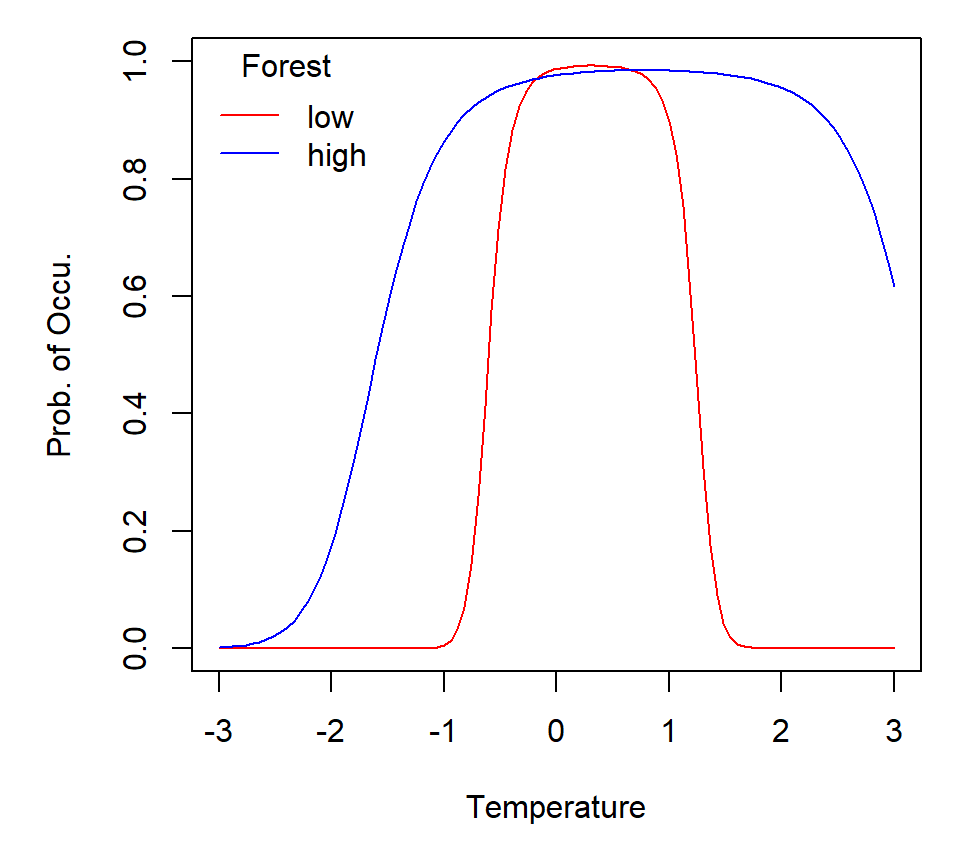## Predict the distribution of lion beetles library(boot) load('c:/A. UFL/rmeetup/est.RData') load('c:/A. UFL/2. Alberta/data/original/EnvData.km2.RData') pi <- inv.logit(est[1,1] + est[2,1] * cov$MAT + est[4,1] * cov$MAT ^ 2 + est[3,1] * cov$forest + est[5,1] * cov$MAT * cov$forest +
est[6,1] * cov$MAT ^ 2 * cov$forest)
pi.scale <- round((pi - min(pi)) / (max(pi) - min(pi)) * 9) + 1

par(mfrow=c(1,1))
par(mar=c(0,0,1,0))
par(oma=c(1,1,1,1))

plot(cov$lat ~ cov$lon, pch=19, cex=.01, axes=F,
xlab='', ylab='',
col=rev(heat.colors(10))[pi.scale])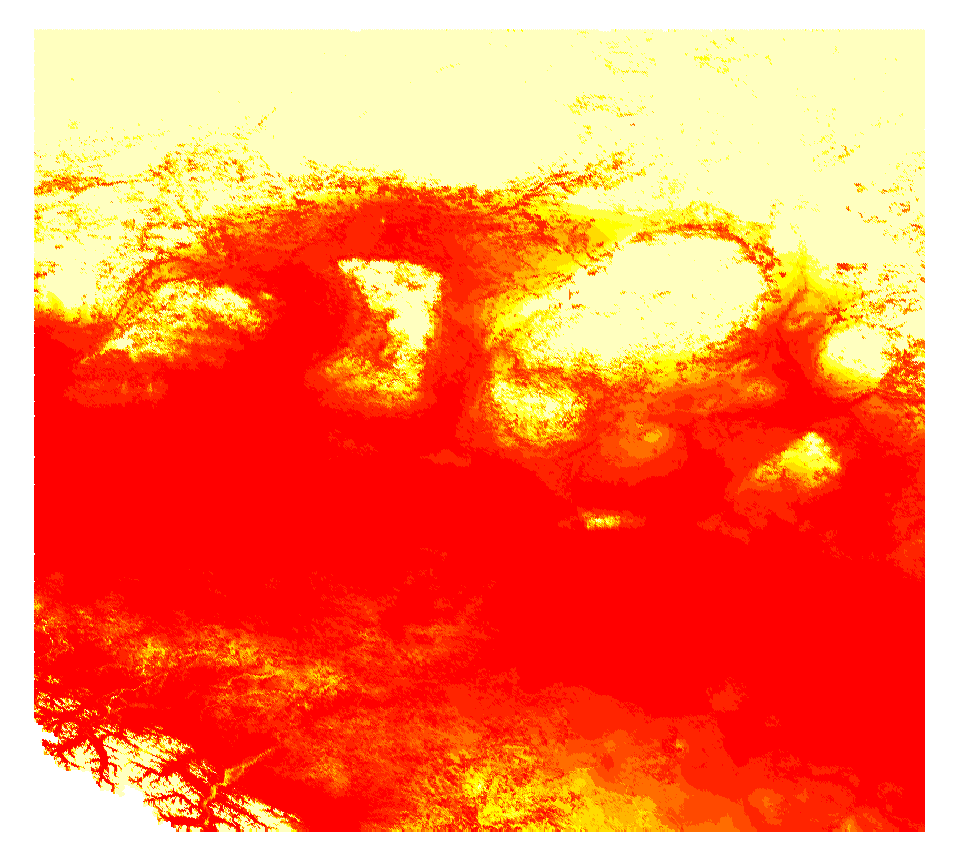## What did occupancy do for us?

• Estimate detection probability
• Explain beetle-environment relationships
• Predict beetle distribution

• Stacked Species Distribution Modelling
• https://cran.r-project.org/web/packages/SSDM/SSDM.pdf
• You can use this package to ensemble a number of machine learning approaches such as Generalized additive model (GAM), Multivariate adaptive regression splines (MARS), Generalized boosted regressions model (GBM), Classification tree analysis (CTA), Random forest (RF), Maximum entropy (MAXENT), Artificial neural network (ANN), and Support vector machines (SVM)

• Multi-species modeling
• Interspecific similarity & undirectional interspecific interactions
• Ovaskainen, O., Tikhonov, G., Norberg, A., Guillaume Blanchet, F., Duan, L., Dunson, D., Roslin, T. and Abrego, N., 2017. How to make more out of community data? A conceptual framework and its implementation as models and software. Ecology Letters, 20(5), pp.561-576.
• Uni-directional interspecific interactions
• Waddle, J.H., Dorazio, R.M., Walls, S.C., Rice, K.G., Beauchamp, J., Schuman, M.J. and Mazzotti, F.J., 2010. A new parameterization for estimating co-occurrence of interacting species. Ecological Applications, 20(5), pp.1467-1475.

• Spatial autocorrelation
• Reviews
• Dormann et al., 2007. Methods to account for spatial autocorrelation in the analysis of species distributional data: a review. Ecography, 30(5), pp.609-628.
• Beale et al., 2010. Regression analysis of spatial data. Ecology letters, 13(2), pp.246-264.
• Crase, B., Liedloff, A.C. and Wintle, B.A., 2012. A new method for dealing with residual spatial autocorrelation in species distribution models. Ecography, 35(10), pp.879-888.
• Hodges, J.S. and Reich, B.J., 2010. Adding spatially-correlated errors can mess up the fixed effect you love. The American Statistician, 64(4), pp.325-334.